$$\gdef\badDispatch#1{\textbf{\textcolor{e1432d}{#1}}} \gdef\noKatexForm#1{\badDispatch{#1}} \gdef\largeDot{\raisebox{0.06em}{\tiny∙}} \gdef\rarrbar{ {\raisebox{-0.05em}{→}\mkern{-0.13em}{\large\shortmid}}} \gdef\larrbar{ { {\large\shortmid}\mkern{-0.13em}{\raisebox{-0.05em}{←}}}} \gdef\suptrans{^\mathsf{T}} \gdef\supdagger{^\dagger} \gdef\rawsymbol#1{\operatorname{#1}} \gdef\colorsymbol#1#2{\textcolor{#1}{\rawsymbol{#2}}} \gdef\lsymbol#1{\colorsymbol{262626}{#1}} \gdef\msymbol#1{\colorsymbol{b50800}{#1}} \gdef\osymbol#1{\colorsymbol{00427f}{#1}} \gdef\lstring#1{\textcolor{666666}{\textrm{\textquotedblleft{\text{#1}}\textquotedblright}}} \gdef\boldForm#1{\textbf{#1}} \gdef\lrform#1{ {\textcolor{e1432d}{#1}}} \gdef\lgform#1{ {\textcolor{4ea82a}{#1}}} \gdef\lbform#1{ {\textcolor{3e81c3}{#1}}} \gdef\lrgform#1{ {\textcolor{ffc87d}{#1}}} \gdef\lgbform#1{ {\textcolor{96e2e3}{#1}}} \gdef\lrbform#1{ {\textcolor{f989b8}{#1}}} \gdef\rform#1{ {\textcolor{cb2b1a}{#1}}} \gdef\gform#1{ {\textcolor{399318}{#1}}} \gdef\bform#1{ {\textcolor{2b6fb0}{#1}}} \gdef\drform#1{ {\textcolor{9b0000}{#1}}} \gdef\dgform#1{ {\textcolor{006800}{#1}}} \gdef\dbform#1{ {\textcolor{004987}{#1}}} \gdef\drgform#1{ {\textcolor{ae5900}{#1}}} \gdef\dgbform#1{ {\textcolor{006567}{#1}}} \gdef\drbform#1{ {\textcolor{86004d}{#1}}} \gdef\rgform#1{ {\textcolor{dc841a}{#1}}} \gdef\gbform#1{ {\textcolor{47a5a7}{#1}}} \gdef\rbform#1{ {\textcolor{c74883}{#1}}} \gdef\pform#1{ {\textcolor{665996}{#1}}} \gdef\dpform#1{ {\textcolor{41326b}{#1}}} \gdef\waform#1{ {\textcolor{6b6b6b}{#1}}} \gdef\wbform#1{ {\textcolor{929292}{#1}}} \gdef\wcform#1{ {\textcolor{c5c5c5}{#1}}} \gdef\tick{\checkmark} \gdef\barToken{\mathbf{|}} \gdef\filledRectangleToken{▮} \gdef\emptyRectangleToken{▯} \gdef\filledSquareToken{■} \gdef\emptySquareToken{□} \gdef\filledToken{∙} \gdef\emptyToken{∘} \gdef\cardinalRewrite#1#2{\rewrite{#1}{#2}} \gdef\primed#1{ {#1}^{\prime}} \gdef\tinybullet{ {\tiny●}} \gdef\factsym#1{\mathord{#1}} \gdef\forwardFactor{\factsym{\uparrow}} \gdef\backwardFactor{\factsym{\downarrow}} \gdef\forwardBackwardFactor{\factsym{\updownarrow}} \gdef\forwardBackwardNeutralFactor{\factsym{\mathrlap{\downarrow}{\mathrlap{\uparrow}{\endash} } }} \gdef\neutralFactor{\factsym{\shornarrow}} \gdef\arrowhead{⏵} \gdef\inverseArrowhead{⏴} \gdef\rightArrowhead{⏵} \gdef\leftArrowhead{⏴} \gdef\upArrowhead{⏶} \gdef\downArrowhead{⏷} \gdef\de#1#2{ { {#1}\xundirectededge{}{#2}}} \gdef\ue#1#2{ { {#1}\xrightedge{}{#2}}} \gdef\shifttag#1{\raisebox{-1em}{#1}} \gdef\shifttag#1{#1} \gdef\tde#1#2#3{ {#1}\:\xrightedge{\shifttag{#3}}\;{#2}} \gdef\tue#1#2#3{ {#1}\;\xundirectededge{\shifttag{#3}}\;{#2}} \gdef\shiftunderset#1#2{\underset{\raisebox{0.15em}{\scriptsize #1}}{#2}} \gdef\mdtde#1#2#3#4{ {#1}\,\;\shiftunderset{#4}{\xrightedge{#3}}\;{#2}} \gdef\mtue#1#2#3#4{ {#1}\,\;\shiftunderset{#4}{\xundirectededge{#3}}\;\,{#2}} \gdef\mtde#1#2#3#4{ {#1}\,\;\operatornamewithlimits{\xrightedge{#3}}\limits_{#4} \;{#2}} \gdef\mapsfrom{\htmlClass{hreflect}{\mapsto}} \gdef\longmapsfrom{\htmlClass{hreflect}{\longmapsto}} \gdef\diffd{𝕕} \gdef\partialdof#1{\partial {#1}} \gdef\emptySet{\empty} \gdef\unknown{\wbform{\text{?}}} \gdef\notApplicable{\wbform{\text{---}}} \gdef\textAnd{\text{\,and\,}} \gdef\identicallyEqualSymbol{\equiv} \gdef\congruentSymbol{\equiv} \gdef\isomorphicSymbol{\simeq} \gdef\homeomorphicSymbol{\cong} \gdef\homotopicSymbol{\simeq} \gdef\approxEqualSymbol{\approx} \gdef\bijectiveSymbol{\approx} \gdef\defeq{\mathrel{≝}} \gdef\defEqualSymbol{\mathrel{≝}} \gdef\syntaxEqualSymbol{\mathrel{\textcolor{888888}{\equiv}}} \gdef\tailEqualSymbol{\underdot{=}} \gdef\headEqualSymbol{\dot{=}} \gdef\ruledelayed{:\to} \gdef\mathdollar{\text{\textdollar}} \gdef\hyphen{\text{-}} \gdef\endash{\text{--}} \gdef\emdash{\text{---}} \gdef\updownarrows{\uparrow\!\downarrow} \gdef\vthinspace{\mkern{1pt}} \gdef\dlq{\text{\textquotedblleft}} \gdef\drq{\text{\textquotedblright}} \gdef\dprime{ {\prime\prime}} \gdef\inverse#1{ { {#1}^{-1}}} \gdef\inverseSymbol{\inverse{□}} \gdef\groupDirectProduct#1{#1} \gdef\groupDirectProductSymbol{\times} \gdef\groupInverse{\inverse} \gdef\groupPower#1#2{ {#1}^{#2}} \gdef\groupCommutator#1#2{\left[{#1},{#2}\right]} \gdef\groupoidInverse{\inverse} \gdef\latticeBFS#1{\textrm{bfs}({#1})} \gdef\pathHomomorphism#1{#1} \gdef\groupoidFunction#1{#1} \gdef\groupoidHomomorphism#1{#1} \gdef\affineModifier#1{\overrightharpoon{#1}} \gdef\supercirc#1{#1^{\circ}} \gdef\supercircb#1{#1^{\bullet}} \gdef\blackCircleModifier#1{\supercircb{#1}} \gdef\whiteCircleModifier#1{\supercirc{#1}} \gdef\totalSpaceStyle#1{\rbform{#1}} \gdef\baseSpaceStyle#1{\bform{#1}} \gdef\fiberSpaceStyle#1{\rform{#1}} \gdef\totalSpaceElementStyle#1{\drbform{#1}} \gdef\baseSpaceElementStyle#1{\dbform{#1}} \gdef\fiberSpaceElementStyle#1{\drform{#1}} \gdef\bundleProjectionStyle#1{\dgform{#1}} \gdef\bundleGraphStyle#1{\rbform{#1}} \gdef\bundleSectionStyle#1{\drgform{#1}} \gdef\bundleFunctionStyle#1{\drbform{#1}} \gdef\graphFunctionStyle#1{\totalSpaceStyle{#1}} \gdef\projectionFunctionStyle#1{\totalSpaceStyle{#1}} \gdef\sectionFunctionStyle#1{\totalSpaceStyle{#1}} \gdef\functionGraph#1{G_{#1}} \gdef\toroidalModifier#1{\supercirc{#1}} \gdef\modulo#1{\supercirc{#1}} \gdef\dividesSymbol{\mathrel{|}} \gdef\groupFunction#1{#1} \gdef\groupHomomorphism#1{#1} \gdef\automorphisms{\operatorname{Aut}} \gdef\endomorphisms{\operatorname{End}} \gdef\transportMap#1{\transportMapSymbol_{#1}} \gdef\transportMapSymbol{\tau} \gdef\action#1{#1} \gdef\selfAction#1{\hat{#1}} \gdef\actionGroupoid#1{\utilde{#1}} \gdef\cardinalGroup#1{G^*({#1})} \gdef\signed#1{ {#1}^*} \gdef\transportAtlas#1{T_{#1}} \gdef\inverted#1{\underline{#1}} \gdef\mirror#1{\overline{#1}} \gdef\pathComposeSymbol{\mathbin{∷}} \gdef\pathCompose#1#2{ {#1}\pathComposeSymbol{#2}} \gdef\translateSymbol{\mathbin{\uparrow}} \gdef\backwardTranslateSymbol{\mathbin{\downarrow}} \gdef\pathTranslate#1#2{ {#1}\translateSymbol{#2}} \gdef\pathLeftTranslate#1{ { {#1}\translateSymbol}} \gdef\pathBackwardTranslate#1#2{ {#1}{\backwardTranslateSymbol}{#2}} \gdef\pathLeftBackwardTranslate#1{ {#1}{\backwardTranslateSymbol}} \gdef\compactCovariantDifference#1#2{\Delta_{#1}\,{#2}} \gdef\covariantDifference{ {#1}\,\Delta\,{#2}} \gdef\forwardDifference{\Delta^{+}} \gdef\backwardDifference{\Delta^{-}} \gdef\centralDifference{\Delta} \gdef\pathForwardDifference#1{\forwardDifference_{#1}} \gdef\pathBackwardDifference#1{\backwardDifference_{#1}} \gdef\pathCentralDifference#1{\centralDifference_{#1}} \gdef\pathHead#1{\pathHeadVector{#1}} \gdef\pathTail#1{\pathTailVector{#1}} \gdef\pathHeadVector#1{ {#1}^{\bullet}} \gdef\pathTailVector#1{ {#1}_{\bullet}} \gdef\pathReverse#1{ {#1}^{\dagger}} \gdef\pathIntegral#1#2{ {#1} \int {#2}} \gdef\pathIntegralSymbol{ {\int}} \gdef\pathDot#1#2{ {#1} \cdot {#2}} \gdef\pathDotSymbol{ {\cdot}} \gdef\compactBasis#1{\mathscr{B}} \gdef\length{\operatorname{len}} \gdef\signedLength{\operatorname{len^*}} \gdef\andFn{\operatorname{and}} \gdef\orFn{\operatorname{or}} \gdef\notFn{\operatorname{not}} \gdef\vertexList{\operatorname{vertices}} \gdef\vertexList{\operatorname{vertices}} \gdef\edgeList{\operatorname{edges}} \gdef\pathList{\operatorname{paths}} \gdef\cardinalList{\operatorname{cards}} \gdef\signedCardinalList{\operatorname{cards^*}} \gdef\wordOf{\operatorname{word}} \gdef\headVertex{\operatorname{head}} \gdef\tailVertex{\operatorname{tail}} \gdef\basis{\operatorname{basis}} \gdef\split{\operatorname{split}} \gdef\lcm{\operatorname{lcm}} \gdef\minimalContractionSets{\operatorname{MCSets}} \gdef\minimalContractions{\operatorname{MC}} \gdef\grade{\operatorname{grade}} \gdef\support{\operatorname{support}} \gdef\coefficient{\operatorname{coeff}} \gdef\domain{\operatorname{domain}} \gdef\codomain{\operatorname{codomain}} \gdef\modFunction{\operatorname{mod}} \gdef\clip{\operatorname{clip}} \gdef\sign{\operatorname{sign}} \gdef\step{\operatorname{step}} \gdef\projection{\operatorname{proj}} \gdef\lift{\operatorname{lift}} \gdef\identity{\operatorname{id}} \gdef\total{\operatorname{total}} \gdef\torus{\operatorname{torus}} \gdef\mobius{\operatorname{mobius}} \gdef\stateCompose{\operatorname{glue}} \gdef\infixStateComposeSymbol{\_} \gdef\stateDecompose{\operatorname{melt}} \gdef\stateJoin{\operatorname{conj}} \gdef\stateMeet{\operatorname{disj}} \gdef\stateExtent{\operatorname{extent}} \gdef\stateIntent{\operatorname{intent}} \gdef\infixStateJoinSymbol{\sqcup} \gdef\infixStateMeetSymbol{\sqcap} \gdef\isPrime#1{#1\textrm{ prime}} \gdef\blank{\_} \gdef\emptyWord{} \gdef\multiwordSymbol#1{\mathbf{#1}} \gdef\wordSymbol#1{\mathtt{#1}} \gdef\word#1{#1} \gdef\pathMap#1{#1} \gdef\function#1{#1} \gdef\imageModifier#1{ {#1}^{\rightarrow}} \gdef\preimageModifier#1{ {#1}^{\leftarrow}} \gdef\multiImageColorModifier#1{\msetCol{#1}} \gdef\multiImageModifier#1{ {#1}^{\Rightarrow}} \gdef\multiPreimageModifier#1{ {#1}^{\Leftarrow}} \gdef\functionComposition#1{#1} \gdef\functionCompositionSymbol{\mathbin{\small ∘}} \gdef\route#1#2#3{[{#1}\!:\!{#2}\!:\!{#3}]} \gdef\multiroute#1#2#3{[{#1}\!:\!{#2}\!:\!{#3}]} \gdef\pathWord#1#2#3{ {#1}\!:\!{#2}\!:\!{#3}} \gdef\parenPathWord#1#2#3{\left(\pathWord{#1}{#2}{#3}\right)} \gdef\nullPath{\bot} \gdef\nullElement{\bot} \gdef\path#1{#1} \gdef\vert#1{#1} \gdef\underdot#1{\underset{\raisebox{0.3em}{.}}{#1}} \gdef\headVertexSymbol{◨} \gdef\tailVertexSymbol{◧} \gdef\placeholderVertexSymbol{\mathrlap{◨}{◧}} \gdef\tvert#1{\underdot{#1}} \gdef\hvert#1{\dot{#1}} \gdef\edge#1{#1} \gdef\card#1{\mathtt{#1}} \gdef\path#1{#1} \gdef\quiver#1{#1} \gdef\bindingRuleSymbol{\to} \gdef\compactBindingRuleSymbol{:} \gdef\cayleyQuiverSymbol#1{\selfAction{#1}} \gdef\bindCayleyQuiver#1#2{\selfAction{#1}[#2]} \gdef\bindActionQuiver#1#2{#1[#2]} \gdef\bindSize#1#2{#1(#2)} \gdef\bindCardSize#1#2{#1[#2]} \gdef\bindCards#1#2{#1[#2]} \gdef\subSize#1#2{#1_{#2}} \gdef\gridQuiver#1{\textrm{Grid}^{#1}} \gdef\treeQuiver#1{\textrm{Tree}^{#1}} \gdef\bouquetQuiver#1{\textrm{Bq}^{#1}} \gdef\lineQuiver{\textrm{L}} \gdef\cycleQuiver{\textrm{C}} \gdef\squareQuiver{\textrm{Sq}} \gdef\cubicQuiver{\textrm{Cbc}} \gdef\triangularQuiver{\textrm{Tri}} \gdef\hexagonalQuiver{\textrm{Hex}} \gdef\rhombilleQuiver{\textrm{Rmb}} \gdef\limit#1#2{\lim_{#2}\,#1} \gdef\realVectorSpace#1{\mathbb{R}^{#1}} \gdef\complexVectorSpace#1{\mathbb{C}^{#1}} \gdef\matrixRing#1#2{M_{#2}(#1)} \gdef\groupoid#1{#1} \gdef\group#1{#1} \gdef\field#1{#1} \gdef\ring#1{#1} \gdef\variable#1{#1} \gdef\semiring#1{#1} \gdef\sym#1{#1} \gdef\matrix#1{#1} \gdef\polynomial#1{#1} \gdef\setLetter{\mathcal{S}} \gdef\signedSetLetter{\mathcal{S^*}} \gdef\multisetLetter{\mathcal{M}} \gdef\signedMultisetLetter{\mathcal{M^*}} \gdef\multisetSemiringSymbol#1{#1} \gdef\multisetSemiring#1#2{\multisetLetter\left[#1, #2\right]} \gdef\signedMultisetRingSymbol#1{#1} \gdef\signedMultisetRing#1#2{\signedMultisetLetter\left[#1, #2\right]} \gdef\polynomialRing#1#2{#1[{#2}]} \gdef\routeSymbol#1{#1} \gdef\multirouteSymbol#1{\mathbf{#1}} \gdef\planSymbol#1{\mathbf{#1}} \gdef\ringElement#1{#1} \gdef\matrixPart#1#2#3{#1\llbracket{#2,#3}\rrbracket} \gdef\matrixRowPart#1{#1} \gdef\matrixColumnPart#1{#1} \gdef\subMatrixPart#1#2#3{#1_{#2,#3}} \gdef\matrixDotSymbol{\cdot} \gdef\matrixPlusSymbol{+} \gdef\wordGroup#1{\wordGroupSymbol_{#1}} \gdef\wordGroupSymbol{\Omega} \gdef\wordRing#1{\wordRingSymbol_{#1}} \gdef\wordRingSymbol{\Omega\!\degree} \gdef\wordRingElement#1{#1} \gdef\wordRingBasisElement#1{e_{#1}} \gdef\linearCombinationCoefficient#1{ {\textcolor{888888}{#1}}} \gdef\plan#1{#1} \gdef\planRing#1{\planRingSymbol_{#1}} \gdef\planRingSymbol{\Phi} \gdef\basisPath#1#2{\mathbf{#1}_{#2}} \gdef\basisPathWeight#1#2{ {#1}_{#2}} \gdef\unitSymbol{\mathbf{e}} \gdef\unitVertexField{\unitSymbol_1} \gdef\forwardSymbol{f} \gdef\backwardSymbol{b} \gdef\symmetricSymbol{s} \gdef\antisymmetricSymbol{a} \gdef\wordVector#1#2{\unitSymbol_{#1}^{#2}} \gdef\gradOf#1{\grad\,{#1}} \gdef\grad{\nabla} \gdef\divOf#1{\div\,{#1}} \gdef\div{\dot{\nabla}} \gdef\laplacianOf#1{\laplacian\,{#1}} \gdef\laplacian{\ddot{\nabla}} \gdef\suchThat#1#2{ {#1}\,\big|\,{#2}} \gdef\chart#1{\chartSymbol_{#1}} \gdef\chartSymbol{C} \gdef\graphRegionIntersectionSymbol{\cap} \gdef\graphRegionUnionSymbol{\cup} \gdef\pathIso{\simeq} \gdef\factorial#1{#1!} \gdef\power#1#2{ {#1}^{#2}} \gdef\repeatedPower#1#2{ {#1}^{#2}} \gdef\kroneckerDeltaForm#1{\kroneckerDeltaSymbol_{#1}} \gdef\kroneckerDeltaSymbol{\delta} \gdef\contractionLattice#1{\operatorname{Con}(#1)} \gdef\contractedRelation#1{\sim_{#1}} \gdef\isContracted#1#2{ {#1} \sim {#2}} \gdef\isContractedIn#1#2#3{ {#1} \sim_{#3} {#2}} \gdef\isNotContracted#1#2{ {#1} \not \sim {#2}} \gdef\isNotContractedIn#1#2#3{ {#1} \not \sim_{#3} {#2}} \gdef\contractionSum#1{#1} \gdef\contractionSumSymbol{\sqcup} \gdef\contractionProduct#1{#1} \gdef\contractionProductSymbol{ {\cdot}} \gdef\graph#1{#1} \gdef\graphHomomorphism#1{#1} \gdef\coversSymbol{\sqsupseteq} \gdef\coveredBySymbol{\sqsubseteq} \gdef\strictlyCoversSymbol{\sqsupset} \gdef\strictlyCoveredBySymbol{\sqsubset} \gdef\graphCovering#1#2#3{ {#2} \sqsupseteq_{#1} {#3}} \gdef\quiverCovering#1#2#3{ {#2} \sqsupseteq^{#1} {#3}} \gdef\powerSetSymbol{\mathcal{P}} \gdef\powerSet#1{\powerSetSymbol({#1})} \gdef\existsForm#1#2{\exists\,{#1}\,:\,{#2}} \gdef\forAllForm#1#2{\forall\,{#1}\,:\,{#2}} \gdef\notted#1{\notSymbol {#1}} \gdef\andSymbol{\land} \gdef\orSymbol{\lor} \gdef\notSymbol{\lnot} \gdef\latticeSymbol#1{#1} \gdef\meetSemilatticeSymbol#1{#1} \gdef\joinSemilatticeSymbol#1{#1} \gdef\posetSymbol#1{#1} \gdef\latticeElementSymbol#1{#1} \gdef\meetSemilatticeElementSymbol#1{#1} \gdef\joinSemilatticeElementSymbol#1{#1} \gdef\posetElementSymbol#1{#1} \gdef\latticeMeetSymbol{\wedge} \gdef\latticeJoinSymbol{\vee} \gdef\latticeTop{\top} \gdef\latticeBottom{\bot} \gdef\semilatticeMeetSymbol{\wedge} \gdef\semilatticeJoinSymbol{\vee} \gdef\semilatticeTop{\top} \gdef\semilatticeBottom{\bot} \gdef\semilatticeSemimeetSymbol{\wedge} \gdef\semilatticeSemijoinSymbol{\vee} \gdef\latticeGreaterSymbol{>} \gdef\latticeGreaterEqualSymbol{\ge} \gdef\latticeLessSymbol{<} \gdef\latticeLessEqualSymbol{\le} \gdef\posetGreaterSymbol{>} \gdef\posetGreaterEqualSymbol{\ge} \gdef\posetLessSymbol{<} \gdef\posetLessEqualSymbol{\le} \gdef\posetCoversSymbol{⋗} \gdef\posetCoveredBySymbol{⋖} \gdef\grpname#1{\operatorname{\mathsf{#1}}} \gdef\mkg#1#2#3{\grpname{#1}({#2},{#3})} \gdef\mka#1#2#3{\mathfrak{#1}({#2},{#3})} \gdef\generalLinearAlgebra#1#2{\mka{gl}{#1}{#2}} \gdef\generalLinearGroup#1#2{\mkg{GL}{#1}{#2}} \gdef\specialLinearAlgebra#1#2{\mka{sl}{#1}{#2}} \gdef\specialLinearGroup#1#2{\mkg{SL}{#1}{#2}} \gdef\projectiveGeneralLinearAlgebra#1#2{\mka{pgl}{#1}{#2}} \gdef\projectiveGeneralLinearGroup#1#2{\mkg{PGL}{#1}{#2}} \gdef\projectiveSpecialLinearAlgebra#1#2{\mka{psl}{#1}{#2}} \gdef\projectiveSpecialLinearGroup#1#2{\mkg{PSL}{#1}{#2}} \gdef\orthogonalAlgebra#1#2{\mka{o}{#1}{#2}} \gdef\orthogonalGroup#1#2{\mkg{O}{#1}{#2}} \gdef\specialOrthogonalAlgebra#1#2{\mka{so}{#1}{#2}} \gdef\specialOrthogonalGroup#1#2{\mkg{SO}{#1}{#2}} \gdef\unitaryAlgebra#1{\mka{u}{#1}{#2}} \gdef\unitaryGroup#1{\mkg{U}{#1}{#2}} \gdef\specialUnitaryAlgebra#1#2{\mka{su}{#1}{#2}} \gdef\specialUnitaryGroup#1#2{\mkg{su}{#1}{#2}} \gdef\spinAlgebra#1#2{\mka{spin}{#1}{#2}} \gdef\spinGroup#1#2{\mkg{Spin}{#1}{#2}} \gdef\pinAlgebra#1#2{\mka{pin}{#1}{#2}} \gdef\pinGroup#1#2{\mkg{Pin}{#1}{#2}} \gdef\symmetricGroup#1{\grpname{Sym}({#1})} \gdef\presentation#1{#1} \gdef\groupPresentation#1#2{\left\langle\,{#1}\,\,\middle|\mathstrut\,\,{#2}\,\right\rangle} \gdef\groupRelationIso{=} \gdef\groupGenerator#1{#1} \gdef\groupRelator#1{#1} \gdef\groupElement#1{#1} \gdef\identityElement#1{#1} \gdef\groupoidElement#1{#1} \gdef\groupIdentity#1{#1} \gdef\groupoidIdentityElement#1#2{#1_{#2}} \gdef\ringIdentity#1{#1} \gdef\iconstruct#1#2{ {#1}\,\,\middle|{\large\mathstrut}\,\,{#2}} \gdef\setConstructor#1#2{\left\{\,\iconstruct{#1}{#2}\,\right\}} \gdef\multisetConstructor#1#2{\left\{\mkern{-2.3pt}\left|\,\,\iconstruct{#1}{#2}\,\right|\mkern{-2.3pt}\right\}} \gdef\cardinalityConstructor#1#2{\left|\,\iconstruct{#1}{#2}\,\right|} \gdef\setToMultiset#1{ {#1}^\uparrow} \gdef\multisetToSet#1{ {#1}^\downarrow} \gdef\subsets#1{\setLetter({#1})} \gdef\signedSubsets#1{\signedSetLetter({#1})} \gdef\multisets#1{\multisetLetter({#1})} \gdef\signedMultisets#1{\signedMultisetLetter({#1})} \gdef\circleSpaceSymbol{S} \gdef\topologicalSpace#1{#1} \gdef\bundleSection#1{#1} \gdef\bundleProjection#1{#1} \gdef\setSymbol#1{#1} \gdef\signedSetSymbol#1{#1} \gdef\multisetSymbol#1{#1} \gdef\signedMultisetSymbol#1{#1} \gdef\setElementSymbol#1{#1} \gdef\signedSetElementSymbol#1{#1} \gdef\multisetElementSymbol#1{#1} \gdef\signedMultisetElementSymbol#1{#1} \gdef\negated#1{\bar{#1}} \gdef\positiveSignedPart#1{ {#1}^+} \gdef\negativeSignedPart#1{ {#1}^-} \gdef\multisetMultiplicitySymbol{\,\raisebox{.1em}{\small\#}\mkern{.1em}\,} \gdef\signedMultisetMultiplicitySymbol{\,\raisebox{.1em}{\small\#}\mkern{.1em}\,} \gdef\boundMultiplicityFunction#1{ {#1}^{\sharp}} \gdef\boundSignedMultiplicityFunction#1{\operatorname{ {#1}^{\sharp}}} \gdef\constructor#1#2{\left.{#1}\,\,\middle|\mathstrut\,\,{#2}\right.} \gdef\elemOf#1#2{ { {#1} \in {#2} }} \gdef\notElemOf#1#2{ { {#1} \notin {#2} }} \gdef\edgeOf#1#2{ { {#1} {\in}_E {#2} }} \gdef\vertOf#1#2{ { {#1} {\in}_V {#2} }} \gdef\pathOf#1#2{ { {#1} {\in}_P {#2} }} \gdef\pathType#1{\operatorName{path}{#1}} \gdef\vertexType#1{\operatorName{vertex}{#1}} \gdef\edgeType#1{\operatorName{edge}{#1}} \gdef\multisetType#1{\operatorName{mset}{#1}} \gdef\signedMultisetType#1{\operatorName{mset^*}{#1}} \gdef\unitInterval{\mathbb{I}} \gdef\fromTo#1{#1} \gdef\fromToSymbol{\mapsto} \gdef\vertexCountOf#1{|{#1}|} \gdef\vertices#1{ V_{#1} } \gdef\edges#1{ E_{#1} } \gdef\vertexField#1{#1} \gdef\edgeField#1{#1} \gdef\pathVector#1{\mathbf{#1}} \gdef\pathVectorSpace#1{\mathscr{#1}} \gdef\baseField#1{#1} \gdef\finiteField#1{\mathbb{F}_{#1}} \gdef\functionSpace#1#2{#2^{#1}} \gdef\finiteTotalFunctionSpace#1#2{#2^{\sub #1}} \gdef\pathGroupoid#1{ { \Gamma_{#1} }} \gdef\forwardPathQuiver#1#2{ {\overrightharpoon{#1}_{#2}}} \gdef\backwardPathQuiver#1#2{ {\overrightharpoon{#1}^{#2}}} \gdef\pathQuiver#1{ {\overrightharpoon{#1}}} \gdef\mto#1#2{ {#1}\mapsto{#2}} \gdef\mtoSymbol{\mapsto} \gdef\groupWordRewriting#1{\langle{#1}\rangle} \gdef\rewrite#1#2{ {#1}\mapsto{#2}} \gdef\rewritingRule#1#2{ {#1}\mapsto{#2}} \gdef\rewritingSystem#1{\mathcal{#1}} \gdef\multiwayBFS#1{\textrm{bfs}({#1})} \gdef\rewritingStateBinding#1#2{ {\left.{#1}\!\mid\!{#2}\right.}} \gdef\rewritingRuleBinding#1#2{#1{\left[\,{#2}\,\right]}} \gdef\namedSystem#1{\mathtt{#1}} \gdef\genericRewritingSystem{\namedSystem{Sys}} \gdef\stringRewritingSystem{\namedSystem{Str}} \gdef\circularStringRewritingSystem{\namedSystem{CStr}} \gdef\turingMachineRewritingSystem{\namedSystem{TM}} \gdef\cellularAutomatonRewritingSystem{\namedSystem{CA}} \gdef\graphRewritingSystem{\namedSystem{Gr}} \gdef\hypergraphRewritingSystem{\namedSystem{HGr}} \gdef\petriNetRewritingSystem{\namedSystem{Petri}} \gdef\localStates#1{\localStatesSymbol^#1} \gdef\regionalStates#1{\regionalStatesSymbol^#1} \gdef\globalStates#1{\globalStatesSymbol^#1} \gdef\keySubStates#1{\keySubStatesSymbol^#1} \gdef\valueSubStates#1{\valueSubStatesSymbol^#1} \gdef\localState#1#2{#2_{#1}} \gdef\regionalState#1{#1} \gdef\globalState#1{#1} \gdef\keySubState#1{#1} \gdef\valueSubState#1{#1} \gdef\lhsState#1{#1_{L}} \gdef\rhsState#1{#1_{R}} \gdef\rewriteLHSRegionalState#1{#1_{L}} \gdef\rewriteRHSRegionalState#1{#1_{R}} \gdef\regionalStateForm#1{\waform{(}#1\waform{)}} \gdef\invalidRegionalState{\rform{\times}} \gdef\emptyRegionalState{\regionalStateForm{}} \gdef\regionalSubstateSymbol{\sqsubseteq} \gdef\regionalSuperstateSymbol{\sqsupseteq} \gdef\comparableRegionalStatesSymbol{\mathbin{\square}} \gdef\incomparableRegionalStatesSymbol{\mathbin{\boxtimes}} \gdef\namedStateSet#1{\mathbf #1} \gdef\localStatesSymbol{\namedStateSet L} \gdef\regionalStatesSymbol{\namedStateSet R} \gdef\globalStatesSymbol{\namedStateSet G} \gdef\keySubStatesSymbol{\namedStateSet L_K} \gdef\valueSubStatesSymbol{\namedStateSet L_V} \gdef\localStateSymbol#1{#1} \gdef\regionalStateSymbol#1{#1} \gdef\globalStateSymbol#1{#1} \gdef\keySubStateSymbol#1{#1} \gdef\valueSubStateSymbol#1{#1} \gdef\infixComposeLocalStatesSymbol{\_} \gdef\composeLocalStatesSymbol{\operatorname{glue}} \gdef\composeLocalStatesForm#1{\composeLocalStatesSymbol(#1)} \gdef\ncard#1{\card{\inverted{#1}}} \gdef\mcard#1{\card{\mirror{#1}}} \gdef\nmcard#1{\card{\inverted{\mirror{#1}}}} \gdef\assocArray#1{\left\langle {#1} \right\rangle} \gdef\openMultiset{\lBrace} \gdef\closeMultiset{\rBrace} \gdef\set#1{\{ {#1} \}} \gdef\signedSet#1{\{ {#1} \}} \gdef\list#1{\{ {#1} \}} \gdef\multiset#1{\openMultiset {#1} \closeMultiset} \gdef\signedMultiset#1{\openMultiset {#1} \closeMultiset} \gdef\styledSet#1#2{#1\{ {#1} #1\}} \gdef\styledList#1#2{#1\{ {#1} #1\}} \gdef\styledMultiset#1#2{#1\openMultiset {#2} #1\closeMultiset} \gdef\styledSignedMultiset#1#2{\openMultiset {#2} \closeMultiset} \gdef\permutationCycle#1{#1} \gdef\permutationCycleSymbol{\to} \gdef\permutationSet#1{#1} \gdef\permutationSetSymbol{;} \gdef\transposition#1#2{ {#1} \leftrightarrow {#2}} \gdef\tuple#1{( {#1} )} \gdef\concat#1{ {#1} } \gdef\paren#1{\left( {#1} \right)} \gdef\ceiling#1{\lceil{#1}\rceil} \gdef\floor#1{\lfloor{#1}\rfloor} \gdef\translationVector#1{\left[{#1}\right]_{\textrm{T}}} \gdef\quotient#1#2{ {#1} / {#2}} \gdef\compactQuotient#1#2#3{ {#1}\;{\upharpoonright_{#2}}\;{#3}} \gdef\multilineQuotient#1#2{\frac{#1}{#2}} \gdef\idElem#1{e_{#1}} \gdef\minus#1{-{#1}} \gdef\elem{\ \in\ } \gdef\vsp{\mkern{0.4pt}} \gdef\iGmult{\vsp} \gdef\gmult{\vsp\ast\vsp} \gdef\Gmult{\vsp\ast\vsp} \gdef\gdot{\vsp\cdot\vsp} \gdef\gDot{\vsp\mathbin{\largeDot}\vsp} \gdef\mdot{\vsp\cdot\vsp} \gdef\smallblackcirc{\vsp\raisebox{0.15em}{\tiny∙}\vsp} \gdef\smallwhitecirc{\vsp\raisebox{0.15em}{\tiny∘}\vsp} \gdef\sgdot{\mathbin{\smallwhitecirc}} \gdef\srdot{\mathbin{\smallblackcirc}} \gdef\srplus{+} \gdef\ellipsis{\,\wbform{...}\,} \gdef\verticalEllipsis{\vdots} \gdef\appliedRelation#1{#1} \gdef\setUnionSymbol{\cup} \gdef\setIntersectionSymbol{\cap} \gdef\setRelativeComplementSymbol{-} \gdef\msetCol{\textcolor{bb4444}} \gdef\repeatedMultiset#1#2{#1\,#2} \gdef\msrdot{\mathbin{\smallblackcirc}} \gdef\msrplus{+} \gdef\smrdot{\mathbin{\smallblackcirc}} \gdef\smrplus{+} \gdef\dotminus{\mathbin{\dot{-}}} \gdef\dotcap{\mathbin{\dot{\cap}}} \gdef\dotcup{\mathbin{\dot{\cup}}} \gdef\multisetUnionSymbol{\dotcup} \gdef\multisetIntersectionSymbol{\dotcap} \gdef\multisetRelativeComplementSymbol{\dotminus} \gdef\multisetSumSymbol{\dotplus} \gdef\cartesianProductSymbol{\times} \gdef\functionType#1#2{ {#1} \to {#2}} \gdef\functionSignature#1#2#3{ { {#1} : {#2} \to {#3}}} \gdef\partialFunctionSignature#1#2#3{ { {#1} : {#2} \rightharpoonup {#3}}} \gdef\poly#1{#1} \gdef\quiverProdPoly#1{#1} \gdef\quiverProdPower#1#2{#1^{#2}} \gdef\quiverProdTimes#1{#1} \gdef\parenLabeled#1#2{#1\ ({#2})} \gdef\parenRepeated#1#2{\parenLabeled{#1}{ {#2}\text{ times}}} \gdef\underLabeled#1#2{\underbrace{#1}_{#2}} \gdef\underRepeated#1#2{\underbrace{#1}_{#2\text{ times}}} \gdef\overLabeled#1#2{\overbrace{#1}^{#2}} \gdef\overRepeated#1#2{\overbrace{#1}^{#2\text{ times}}} \gdef\modLabeled#1#2{ {#1 }\textrm{ mod }{#2}} \gdef\freeGroup#1{F_{#1}} \gdef\cyclicGroup#1{\mathbb{Z}_{#1}} \gdef\componentSuperQuiverOfSymbol{\succ} \gdef\setCardinality#1{|{#1}|} \gdef\multisetCardinality#1{|{#1}|} \gdef\dependentQuiverProductSymbol{\mathbin{\times}} \gdef\rightIndependentQuiverProductSymbol{\mathbin{⋊}} \gdef\leftIndependentQuiverProductSymbol{\mathbin{⋉}} \gdef\rightStrongQuiverProductSymbol{\mathbin{⧒}} \gdef\leftStrongQuiverProductSymbol{\mathbin{⧑}} \gdef\rightFiberQuiverProductSymbol{\mathbin{⧕}} \gdef\leftFiberQuiverProductSymbol{\mathbin{⧔}} \gdef\lockedQuiverProductSymbol{\mathbin{\searrow}} \gdef\rightFreeQuiverProductSymbol{\mathbin{\smallerthan}} \gdef\leftFreeQuiverProductSymbol{\mathbin{\largerthan}} \gdef\strongIndependentQuiverProductSymbol{\mathbin{⨝}} \gdef\cartesianQuiverProductSymbol{\mathbin{□}} \gdef\strongQuiverProductSymbol{\mathbin{⊠}} \gdef\graphUnionSymbol{\sqcup} \gdef\graphProductSymbol{\times} \gdef\inlineProdSymbol{|} \gdef\serialCardSymbol{ {:}} \gdef\parallelCardSymbol{ {\mid}} \gdef\cardinalSequenceSymbol{ {:}} \gdef\cardinalProduct#1{(#1)} \gdef\vertexProduct#1{(#1)} \gdef\edgeProduct#1{(#1)} \gdef\cardinalProductSymbol{\inlineProdSymbol} \gdef\vertexProductSymbol{\inlineProdSymbol} \gdef\edgeProductSymbol{\inlineProdSymbol} \gdef\verticalVertexProduct#1#2{\cfrac{#1}{#2}} \gdef\verticalCardinalProduct#1#2{\cfrac{#1}{#2}} \gdef\indexSum#1#2#3{ {\sum_{#2}^{#3} #1}} \gdef\indexProd#1#2#3{ {\prod_{#2}^{#3} #1}} \gdef\indexMax#1#2#3{ {\max_{#2}^{#3} #1}} \gdef\indexMin#1#2#3{ {\min_{#2}^{#3} #1}} \gdef\indexUnion#1#2#3{ {\bigcup_{#2}^{#3} #1}} \gdef\indexIntersection#1#2#3{ {\bigcap_{#2}^{#3} #1}} \gdef\indexGraphUnion#1#2#3{ {\bigcup_{#2}^{#3} #1}} \gdef\indexGraphDisjointUnion#1#2#3{ {\bigcup_{#2}^{#3} #1}} \gdef\styledIndexSum#1#2#3#4{ {#1\sum_{#3}^{#4} #2}} \gdef\styledIndexProd#1#2#3#4{ {#1\prod_{#3}^{#4} #2}} \gdef\styledIndexMax#1#2#3#4{ {#1\max_{#3}^{#4} #2}} \gdef\styledIndexMin#1#2#3#4{ {#1\min_{#3}^{#4} #2}} \gdef\indexSumSymbol{\sum} \gdef\indexProdSymbol{\prod} \gdef\indexMaxSymbol{\max} \gdef\indexMinSymbol{\min} \gdef\openInterval#1#2{(#1,#2)} \gdef\closedInterval#1#2{[#1,#2]} \gdef\openClosedInterval#1#2{(#1,#2]} \gdef\closedOpenInterval#1#2{[#1,#2)} \gdef\oneTo#1{1..{#1}} \gdef\zeroTo#1{0..{#1}} \gdef\qstring#1{\mathtt{"}{#1}\mathtt{"}} \gdef\wstring#1{\textcolor{6b6b6b}{#1}} \gdef\qchar#1{\mathtt{'}{#1}\mathtt{'}} \gdef\lstr#1{\mathtt{#1}} \gdef\lchar#1{\mathtt{#1}} \gdef\string#1{ {#1}} \gdef\character#1{ {#1}} \gdef\homomorphismMapping#1{\assocArray{#1}} \gdef\starModifier#1{ {#1}^*} \gdef\translationPresentation#1{\textrm{Z}_{#1}} \gdef\starTranslationPresentation#1{\textrm{Z}^*_{#1}} \gdef\translationPathValuation#1{\mathcal{\overrightharpoon Z}_{#1}} \gdef\starTranslationPathValuation#1{\overrightharpoon{\mathcal{Z}^*_{#1}}} \gdef\translationWordHomomorphism#1{\mathcal{Z}_{#1}} \gdef\starTranslationWordHomomorphism#1{\mathcal{Z}^*_{#1}} \gdef\translationCardinalValuation#1{\textrm{T}_{#1}} \gdef\starTranslationCardinalValuation#1{\textrm{T}^*_{#1}}$$
Noncommutativity

# Noncommutativity

## Introduction

The line, square, triangular, etc. quivers we have examined in Transitive quivers, Intransitive lattices, and Toroidal lattices have all been associated with finite- or infinite-order Abelian groups. Specifically, they could be seen as the Cayley quivers of presentations of these groups, or in for non-transitive quivers like the hexagonal quiver, as path quotients by affine path valuations with values in these groups.

Ultimately, the group associated with each such quiver can be interpreted as the translation group that describes how we can permute the vertices of the quiver, while preserving path words. This translation group is the group center of the endomorphism group of the quiver, which is the larger group of path homomorphisms that permute vertices and rewrite path words in an invertible way.

Let us now move beyond Abelian groups, to non-commutative groups. One family of "minimally non-commutative" groups are the dihedral groups, which describe the symmetries of a n-gon. We'll examine the infinite dihedral groups, which, in the 1-dimensional case, describe symmetries of a "polygon with infinite number of edges".

## Dihedral lattices

Let’s look at the one-dimensional family first. The cardinals $$\rform{\card{x}}$$ and $$\rform{\inverted{\card{x}}}$$ move right and left, and $$\rgform{\card{f}}$$ flips the direction of movement. The matrix representation used is: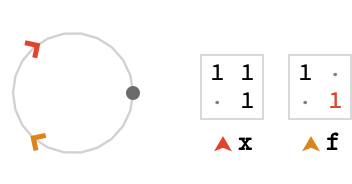The lattice so generated is a doubled version of the line lattice, with the $$\rgform{\card{f}}$$ switching between the upper and lower portions: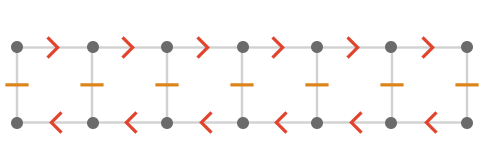We can generalize this concept to the two-dimensional infinite dihedral group, with cardinals $$\rform{\card{x}}$$, $$\bform{\card{y}}$$. This representation is: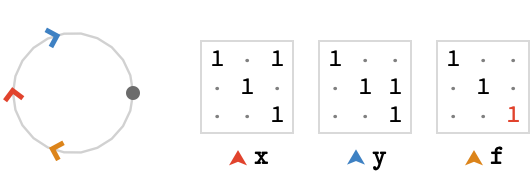The generated lattice consists of two oppositely oriented copies of the square lattice, with the $$\rgform{\card{f}}$$ cardinal acting to alternate between them: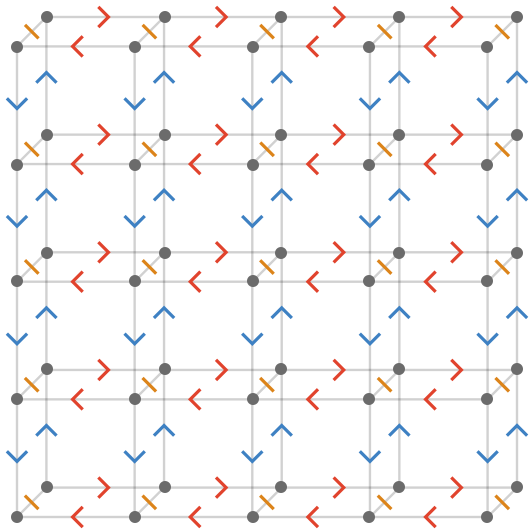## Wreathe product

This immediately suggests a general procedure, since the flip cardinal is effectively a generator of a group action on the other cardinals via $$\list{\rewrite{\rform{\card{x}}}{\rform{\inverted{\card{x}}}},\rewrite{\bform{\card{y}}}{\bform{\inverted{\card{y}}}}}$$. We can therefore take any lattice quiver with an abelian group, and add to it additional cardinals that permute and/or invert its other cardinals. I claim without proof that this is equivalent to forming a restricted wreath product with base group being the "pure translation" cardinals. The infinite dihedral group above is a wreathe product with a group isomorphic to $$\mathbb{Z}_2$$.

As one example of this procedure, let’s add a cardinal $$\gform{\card{r}}$$ that performs a 90° rotation of the other cardinals, which corresponds to taking a wreathe product with a group isomorphic to $$\mathbb{Z}_4$$: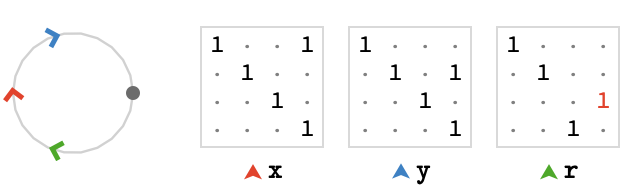The generated lattice produces contains four copies of the square lattice: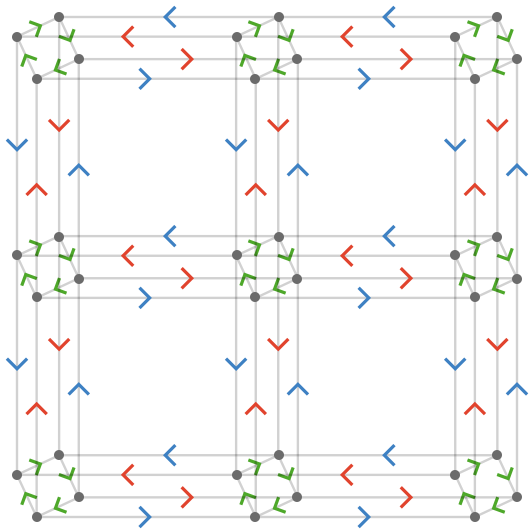Each application of the $$\gform{\card{r}}$$ cardinal cycles us among four different square lattices in which the roles of $$\rform{\card{x}}$$ and $$\bform{\card{y}}$$ are cycled through the list $$\list{\rform{\card{x}},\bform{\card{y}},\rform{\inverted{\card{x}}},\bform{\inverted{\card{y}}}}$$.

Unfortunately the representation I used above only generates a cover of the true lattice quiver. I had to write the cardinal matrices directly in order to produce a tractable coordinatization, but if one abandons matrix representations and uses a finite state machine directly, the lattice can be generated in that fashion quite directly.

A small aside: a famous example of a wreathe group is the “lamplighter group”: an bi-infinite street of lamps, and a lamplighter who can either move left or right, or toggle the lamp he is at. This admits a straightforward state machine implementation. For a “circular street” of $$n$$lamps, we obtain some very symmetric polyhedra: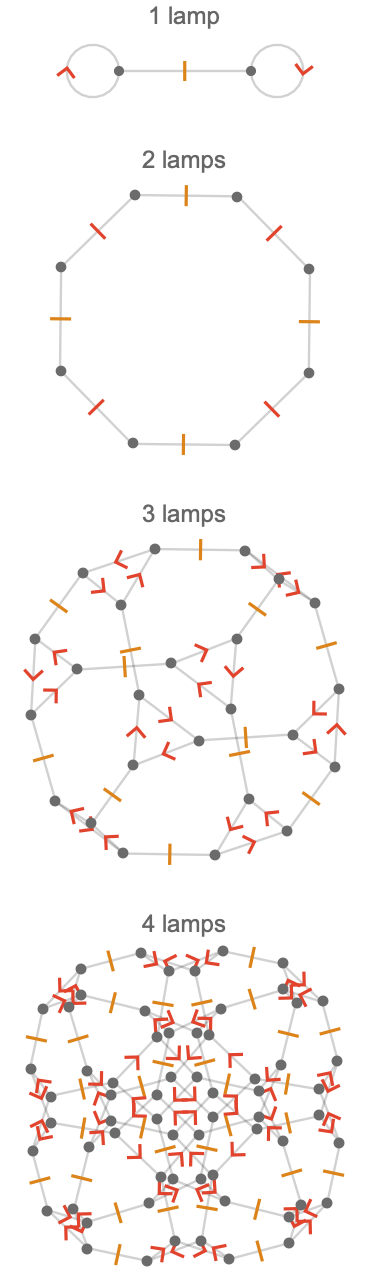One way to understand these polyhedra is that they are truncated hypercubes (this is most obvious visually for the 2 and 3-lamp cases above). The reason for this is simple: the possible configurations of $$n$$ lamps form the vertices of an $$n$$-hypercube, with each axis corresponding to a lamp. Flipping a particular lamp is equivalent to traversing an edge of this hypercube. If we are at a corner of the hypercube, we can flip any lamp we wish since there are $$n$$ edges incident to us. To prevent this, imagine “shaving off” a corner of the $$n$$-hypercube to obtain a polygon with $$n-1$$ sides. Each corner of this polygon has one “toggle” cardinal associated with it, corresponding to toggling a single lamp. The two neighboring polygon corners represent the togglings available if we walk to the next or previous lamp. By walking along this polygon, we can reach any particular lamp in order to flip it.

## Enumerations